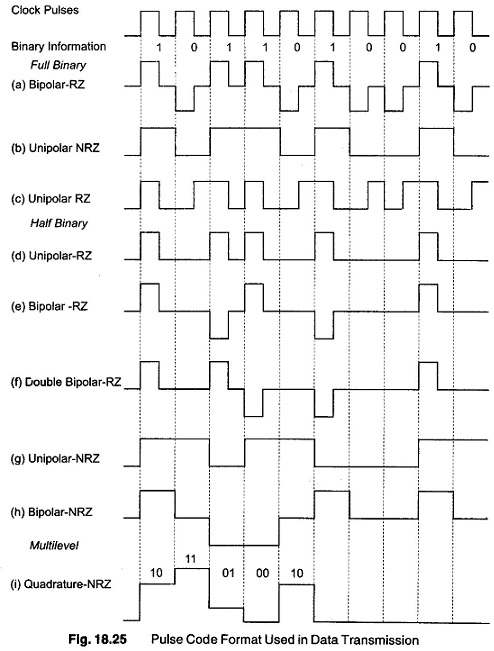## Pulse Wave Code Format:

There are many different code formats of pulse wave. The classification is based on three criteria, namely, form of information transmission, relation to zero level, and direction.

Based on the form of information transmission, the format used can be any one of the following

1. Full binary transmission, where both 0 and 1 bits are part of the format.
2. Half binary transmission, where only the 1 are transmitted. The 0 is recognised by the absence of a pulse at the time of clock transition.
3. Multiple binary transmission, where ternary and quadratic codes are used for each transmitted pulse.

Based on its relationship to the zero level, the transmission format can be either Return to Zero (RZ) in which there is a return to zero level after the transmission of each bit of information, or Non return to Zero (NRZ), where there is no voltage level change if consecutive bits are transmitted, although there is a level change when there is an information variation from 0 to 1 or 1 to 0. In the case of the third criteria, that is, direction, the code format used can be either unipolar, where the Pulse Wave are in a single direction, or bipolar, where the pulses are in both directions. Some of these code formats are shown in Fig. 18.25.### Full Binary Transmission

The full binary bipolar return to zero (RZ) format shown in Fig. 18.25 (a) is one of the most reliable Pulse Wave code formats employed for slow speed transmission. The speed is typically up to 600 bits/s. Using frequency shift keying (FSK), opposite polarity pulses are used to transmit “1” and “0” bits. No power is transmitted between the pulses, resulting in a space between each pulse.

In the non-return to zero (NRZ) unipolar type of transmission, shown in Fig. 18.25(b) the pulses are spread out in time so that they occupy the full time slot and permit an increasing rate of transmission. The term NRZ is directly related to the fact that the Pulse Wave do not return to zero between successive pulses of 0 and 1. This format is most popularly used in serial computer applications and for data transmission speeds of 600, 1200 and 2400 bits/s. The transmission band width is efficiently utilised, since the entire bit period contains signal information.

The RZ unipolar format, shown in Fig. 18.25 (c), has symmetrical format characterised by the absence of a dc level. The Pulse Wave format has a zero crossing for each bit period, a feature that simplifies synchronization, at the expense of increasing the total- band width required. This code is also referred to as a split phase, biphase or Manchester code.

### Half Binary Transmission

In the half binary transmission system, the binary ls are represented by a pulse or a polarity change, but the Os are seen as spaces. This is based on the statistical assumption that the number of is in a pulse train is equal to the number of Os, resulting in a reduction of the transmission power with a possi­ble increase of transmission speed. The RZ unipolar format in half binary transmission is shown in Fig. 18.25 (d). The pulse train is similar to that in Fig. 18.25 (a), except that the Pulse Wave corresponding to the 0 bits are absent. As a result, the frequency spectrum of the pulse train has fewer high frequency components, which in turn results in less cross-talk. However, a dc component also results, which is difficult to transmit. The coding method is not efficient, since 50% of the band width is wasted because information is contained in only half the bit periods.

Scroll to Top